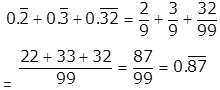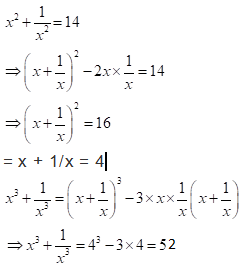# Algebra - Online Test

Q1. If a and b are rational numbers and, then a : b is equal to
Explaination / Solution:

(a + b√3) = [1 / (2 - √3)]
= [(2 + √3) / (2 - √3) (2 + √3)]……..(multiplying numerator and denominator by conjugate of denominator)
= [(2 + √3) / {(2)2 – (√3)2}] …………(x – y) (x + y) = (x2 – y2
= [ (2 + √3) / (4 – 3) ]
= ( 2 + √3)
Ã (a + b√3) = ( 2 + 1√3)
Ã a = 2, and b = 1
Ã a:b = 2:1

Q2. Find the value of a3 + b3 + c3 – 3abc when a = 225, b = 226, c = 227.
Explaination / Solution:

Applying this a3 + b3 + c3 – 3abc = 1/2(a+b+c)[(a-b)2 + (b-c)2 + (c-a)2]
=1/2(225+226+227)*[(225-226)2 + (226-227)2 + (227-225)2]
=1/2(678)*(1+1+4)
=2034

Q3. If 4x = 18y, then the value ofis
Explaination / Solution:

4x = 18y
x/y = 18/4 = 9 /2
so,
(x/y – 1) = 9 /2 – 1 = 7 / 2

Q4. If x = 3+2√2, then solve for √x - 1/√x?
Explaination / Solution:

(√x - 1/√x)2 = x + 1/ x – 2
= (3 + 2√2) + 1 / (3+2√2) – 2
= (3 + 2√2) + 1/ (3+2√2) * (3-2√2)/(3 - 2√2) – 2
= (3 + 2√2) + (3 - 2√2) – 2 = 4
= (√x - 1/√x) = 2

Q5. Which of the following is not is in increasing order.
Explaination / Solution:

if num are same then fraction having bigger deno is lesser than the fraction having smaller deno. so, b is in decreasing order

Q6. Ifthen the value ofis
Explaination / Solution:Q7. The value ofis
Explaination / Solution:Q8. Ifthen the value ofis
Explaination / Solution:Q9. Ifthen the value ofis
Explaination / Solution:=0       as 28-27-1= 0

Q10. If, where x is positive, then find the value ofExplaination / Solution:

(a + b)2 = a2 + b2 + 2ab
(a + b)3 = a3 + b3 + 3ab(a + b)